Importance: Medium ✭✭
 Author(s): Euler, Leonhard P.
 Subject: Number Theory » Computational Number Theory
 Keywords:
Problem   Find six positive integers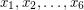such thator prove that such integers do not exist.
Euler's sum of powers conjecture states that forthe Diophantine equationdoes not have solutions in positive integers as soon as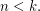For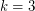it corresponds to a particular case of Fermat Last Theorem and hence is true. For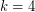and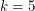, counterexamples to the Euler's sum of powers conjecture were found by N. Elkies in 1986 and L. J. Lander, T. R. Parkin in 1966 respectively. For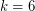, no counterexamples are currently known.